## Example Questions

### Example Question #3 : How To Find The Common Factor Of Square Roots

Which of the following is equivalent to: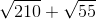?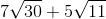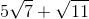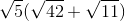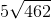Explanation:

To begin with, factor out the contents of the radicals.  This will make answering much easier: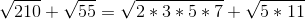They both have a common factor.  This means that you could rewrite your equation like this: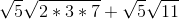This is the same as: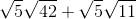These have a common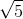.  Therefore, factor that out: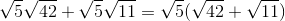### Example Question #4 : How To Find The Common Factor Of Square Roots

Simplify: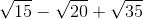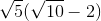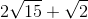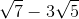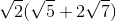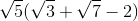Explanation:

These three roots all have ain common; therefore, you can rewrite them:Now, this could be rewritten: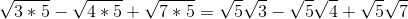Now, note that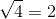Therefore, you can simplify again: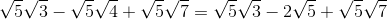Now, that looks messy! Still, if you look carefully, you see that all of your factors have; therefore, factor that out: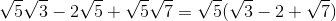This is the same as:### Example Question #1 : How To Find The Common Factor Of Square Roots

Simplify the following: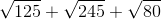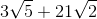It cannot be simplified any further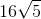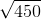Explanation:

Begin by factoring each of the roots to see what can be taken out of each: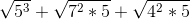These can be rewritten as: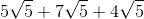Notice that each of these has a common factor of.  Thus, we know that we can rewrite it as: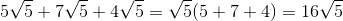### Example Question #2 : How To Find The Common Factor Of Square Roots

Simplify the following: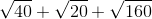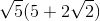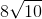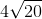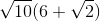The expression cannot be simplified any further.Explanation:

Clearly, all three of these roots have a common factorinside of their radicals. We can start here with our simplification. Therefore, rewrite the radicals like this: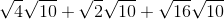We can simplify this a bit further: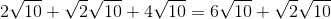From this, we can factor out the common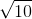: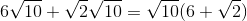### Example Question #3 : Factoring Common Factors Of Squares And Square Roots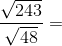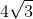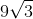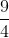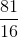Explanation:

To attempt this problem, attempt to simplify the roots of the numerator and denominator: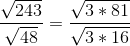Notice how both numerator and denominator have a perfect square: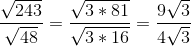The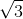term can be eliminated from the numerator and denominator, leaving### Example Question #4 : Factoring Common Factors Of Squares And Square Roots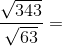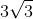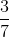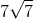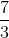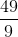Explanation:

For this problem, begin by simplifying the roots. As it stands, numerator and denominator have a common factor ofin the radical: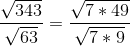And as it stands, thisis multiplied by a perfect square in the numerator and denominator: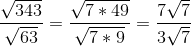The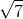term can be eliminated from the top and bottom, leaving### Example Question #31 : Basic Squaring / Square Roots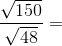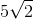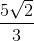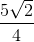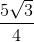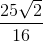Explanation:

To solve this problem, try simplifying the roots by factoring terms; it may be noticeable from observation that both numerator and denominator have a factor ofin the radical: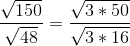We can see that the denominator has a perfect square; now try factoring thein the numerator: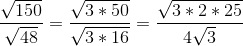We can see that there's a perfect square in the numerator: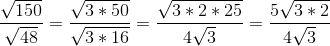Since there is ain the radical in both the numerator and denominator, we can eliminate it, leavingTired of practice problems?

Try live online GRE prep today.